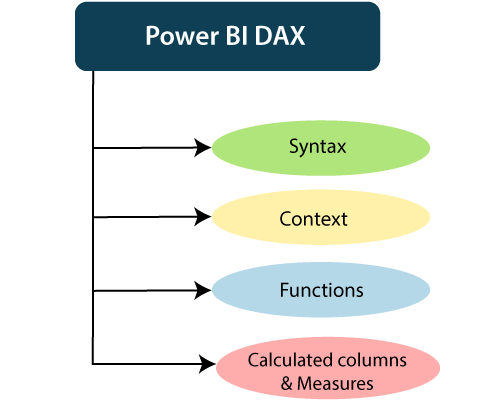# What is Power Bi DAX and How it works?

## Power BI DAX

DAX (Data Analysis Expressions) is a formula expression language. It can be used in different BI and visualization tools. DAX is also known as function language in which the full code is kept inside a function. DAX programming formula contains two data types such as Numeric and Other .

Numeric includes currency, integers, and decimals, where Other includes string and a binary object.### How does it work?

For understanding the Power BI DAX, it has main three fundamental concepts such as:

• Syntax
• Context
• Functions

#### 1. Syntax

the syntax consists of various components that make up a formula.

Total Sales = SUM (Sales [SalesAmount])

• Total Sales is the measure name.
• The equal sign (=) operator indicates the beginning of the formula.
• The DAX function SUM adds up all the numbers in the Sales[SalesAmount] column.
• Parentheses () surround an expression containing one or more arguments. And all function requires at least one argument. An argument passes a value to a function.
• The reference table Sales.
• The referenced column [SalesAmount] in the Sales table. With this argument, the SUM function knows on which column to aggregate a SUM.

#### 2. Context

Context is one of the essential concepts of DAX. It is categorized into two parts; Row context and Filter context.

The Row-Context is the easiest thought of as the current row. It applies whenever a formula has a function which uses the filters to identify a single row in a table.

The Filter context is a little more challenging to understand than the Row context. You can most easily think of the Filter-Context as one or more filters applied in a calculation. The Filter-Context doesn’t exist in the Row-context’s stead. Instead, it uses in addition to the former. Look at the following DAX formula.

#### 3. Functions

Functions are predefined and ordered formula. They can perform calculations using arguments passed on to them. These arguments can be text, numbers, logical values, or other functions.

Types of Functions

Here are some important DAX functions:

1. Aggregate Functions

• MIN
This DAX function returns the minimum numeric value in a column, or between the two scalar expressions.
Syntax

`

`MIN(<column>)`

`

• MAX
This DAX function returns the maximum value in a column, including any logical values and numbers represented as text.
Syntax

` MAX(<column>)`

• AVERAGE
This DAX function returns the arithmetic mean of the values in a column.
Syntax

`

`> AVERAGE(<column>)`

`

• SUM
This DAX function adds all the numbers in a column.
Syntax

`

SUM()

`

2. Count Function

• COUNT
This DAX function is used to return the count of items in a column. If there are multiple numbers of the same thing, this function will count it as separate items and not a single item.
Syntax

`

COUNT()

`

• DISTINCTCOUNT
This DAX function is used to return the distinct count of items in a column. If there are multiple numbers of the same thing, this function will count it as a single item.
Syntax

`

DISTINCTCOUNT()

`

3. Date time Function

• DATE
This DAX function returns the specified date in Date-Time format.
Syntax

`

`> DATE(<year>, <month>, <day>)`

`

• HOUR
This DAX function returns the specified hour as a number from 0 to 23 (12:00 A.M. to 11:00 P.M.).
Syntax

`

HOUR(>datetime<)

`

4. Logical Function

• AND
This DAX function performs logical AND(conjunction) on two expressions. For AND to return true, both conditions specified have to be fulfilled.
Syntax

`

` AND(<logical argument1>,<logical argument2>)`

`

• OR
This DAX function performs logical OR(disjunction) on two expressions. For OR to return true, either of the two conditions specified has to be fulfilled.
Syntax

` OR(<logical argument1>,<logical argument2>)`

• NOT
This DAX function performs logical NOT (negation) on given expression.
Syntax

` NOT(<logical argument>`)

5. Text function

• CONCATENATE
This DAX function joins two text strings into one text string.
Syntax

` CONCATENATE(<text1>, <text2>)`

• FIXED
This DAX function rounds a number to the specified number of decimals and returns the result as text.
Syntax

`FIXED(<number>, <decimals>, <no_commas>)`

• REPLACE
This DAX function replaces part of a text string, based on the number of characters you specify, with a different text string.
Syntax

` REPLACE(<old_text>, <start_num>, <num_chars>, <new_text>)`

### Calculated Columns and Measures

The Power BI DAX formulae are used in calculations, in Measures and Calculated Columns.

Calculated Columns

When you create a data model on the Power BI Desktop, you can extend a table by creating new columns. The content of the columns is defined by a DAX expression, evaluated row by row or in the context of the current row across that table.

Measures

There is another way of defining calculations in a DAX model, useful if you need to operate on aggregate values instead of on a row-by-row basis. These calculations are measures. One of the requirements of DAX is a measure that needs to be defined in a table. However, the action does not belong to the table. So, you can move a measure from one table to another one without losing its functionality.